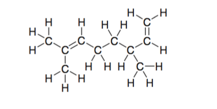# Problem: In Section 1.12 we discussed the effect that branching can have on the boiling point of a compound. In certain instances, branching may also affect how a molecule can react with different molecules. We use the term “steric hindrance” to describe how branching can influence reactivity. For example, greater “steric crowding” may decrease the reactivity of a C=C π bond (Org. Lett. 1999, 1, 1123–1125). In the following molecule, identify each π bond and determine which has a greater degree of steric crowding. (Note: This is not the same as “steric number”.)

###### Problem Details

In Section 1.12 we discussed the effect that branching can have on the boiling point of a compound. In certain instances, branching may also affect how a molecule can react with different molecules. We use the term “steric hindrance” to describe how branching can influence reactivity. For example, greater “steric crowding” may decrease the reactivity of a C=C π bond (Org. Lett. 1999, 1, 1123–1125). In the following molecule, identify each π bond and determine which has a greater degree of steric crowding. (Note: This is not the same as “steric number”.)What scientific concept do you need to know in order to solve this problem?

Our tutors have indicated that to solve this problem you will need to apply the Hybridization concept. You can view video lessons to learn Hybridization. Or if you need more Hybridization practice, you can also practice Hybridization practice problems.

What is the difficulty of this problem?

Our tutors rated the difficulty ofIn Section 1.12 we discussed the effect that branching can h...as medium difficulty.

How long does this problem take to solve?

Our expert Organic tutor, Jonathan took 1 minute and 52 seconds to solve this problem. You can follow their steps in the video explanation above.

What textbook is this problem found in?

Our data indicates that this problem or a close variation was asked in Organic Chemistry - Klein 2nd Edition. You can also practice Organic Chemistry - Klein 2nd Edition practice problems.